# 1) A 12.3 kg particle is undergoing simple harmonic motion with an amplitude of 1.86 mm....

1) A 12.3 kg particle is undergoing simple harmonic motion with an amplitude of 1.86 mm. The maximum acceleration experienced by the particle is 7.93 km/s2. (a) Find the period of the motion. (b) What is the maximum speed of the particle? (c) Calculate the total mechanical energy of this simple harmonic oscillator.

2) The orbit of the Moon around the Earth as projected along a diameter can be viewed as simple harmonic motion. Calculate the effective force constant k for this motion

3) A block of mass M, at rest on a horizontal, frictionless table, is attached to a rigid support by a spring of force constant k. A bullet of mass m and speed v strikes the block as shown in Fig. 17.33. The bullet remains embedded in the block. Determine the amplitude of the resulting simple harmonic motion in terms of m, M, v, and k.

Firstly calculate the angular frequency (w)

Then we can find time period, Vmax and Total Mechanical energy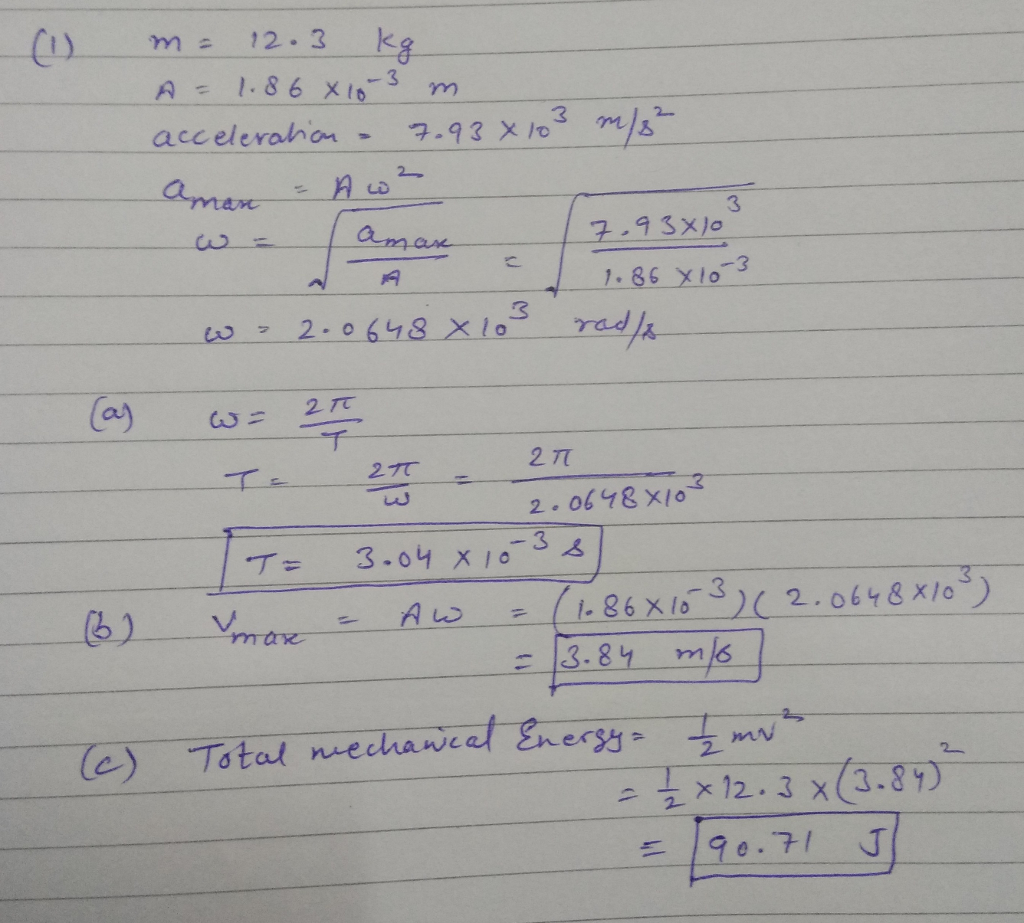#### Earn Coin

Coins can be redeemed for fabulous gifts.

Similar Homework Help Questions
• ### A particle attached to a spring with k = 54 N/m is undergoing simple harmonic motion,...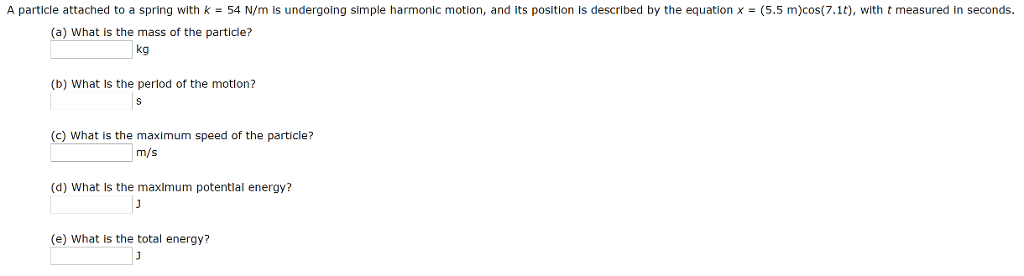A particle attached to a spring with k = 54 N/m is undergoing simple harmonic motion, and its position is described by the equation x = (5.5 m)cos(7.1t), with t measured in seconds (a) What is the mass of the particle? kg (b) What is the perlod of the motion? (c) What is the maximum speed of the particle? m/s (d) What Is the maximum potentlal energy? (e) What is the total energy?

• ### A simple harmonic oscillator consists of a block attached to a spring, moving back and forth...

A simple harmonic oscillator consists of a block attached to a spring, moving back and forth on a frictionless horizontal surface. Suppose the mass of the box is 5.0 kg. The motion is started by holding the box at .50m from its central position, using a force of 40.0 N. Then the box is let go and allowed to perform simple harmonic motion. What is the amplitude of the motion? What is the spring constant k? What is the maximum...

• ### Energy in simple harmonic motion A 2.90 kg object oscillates with simple harmonic motion on a...

Energy in simple harmonic motion A 2.90 kg object oscillates with simple harmonic motion on a spring of force constant 600 N/m. The maximum speed is 0.800 m/s. A) What is the total energy of the object and the spring? B) What is the maximum amplitude of the oscillation?

• ### A particle of mass 5.0 × 10–3 kg, moving with simple harmonic motion of amplitude 0.15...

A particle of mass 5.0 × 10–3 kg, moving with simple harmonic motion of amplitude 0.15 m, takes 47 s to make 50 oscillations. What is the maximum kinetic energy of the particle?

• ### A simple harmonic oscillator consists of a block attached to a spring, moving back and forth...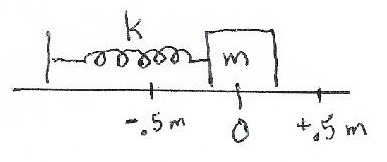A simple harmonic oscillator consists of a block attached to a spring, moving back and forth on a frictionless horizontal surface. Suppose the mass of the box is 5.0 kg. The motion is started by holding the box at 0.50 m from its central position, using a force of 40.0 N. Then the box is let go and allowed to perform simple harmonic motion. (a) What is the amplitude of the motion? (b) What is the spring constant k? (c)...

• ### The maximum velocity of a particle executing simple harmonic motion with amplitude 7.0 mm is 4.4...

The maximum velocity of a particle executing simple harmonic motion with amplitude 7.0 mm is 4.4 m/s. The period of oscillation is?

• ### What is the amplitude of the resulting simple harmonic motion?A 34.0kg block at rest on a horizontal frictionless table is connected to the wall via a spring with a spring constant k=28.0N/m. A 3.90×10-2kg bullettravelling with a speed of 490m/s embeds itself in the block. What is the amplitude of the resulting simple harmonic motion? Recall that the amplitude is themaximum displacement from equilibrium.

• ### Simple Harmonic MotionA block of mass 0.3 kg is attached to a spring of spring constant 28 N/m on a frictionless track. The block moves in simple harmonic motion with amplitude 0.12 m.While passing through the equilibrium point from left to right, the block is struck by a bullet, which stops inside the block. The velocity of the bulletimmediately before it strikes the block is 63 m/s and the mass of the bullet is 1.71 g.The collision occurred at the equilibrium position....

• ### A 0.109-kg particle undergoes simple harmonic motion along the horizontal x-axis between the points ㄨㄧ--0.279 m...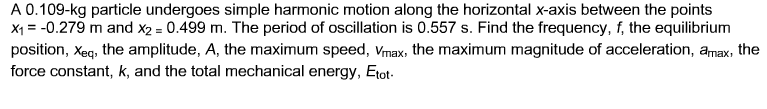A 0.109-kg particle undergoes simple harmonic motion along the horizontal x-axis between the points ㄨㄧ--0.279 m and X2 = 0.499 m. The period of oscillation is 0.557 s. Find the frequency, the equilibrium position, Xeq, the amplitude, A, the maximum speed, Vmax, the maximum magnitude of acceleration, amax, the force constant, k, and the total mechanical energy, Etot

• ### A simple harmonic oscillator consists of a block attached to a spring, moving back and forth...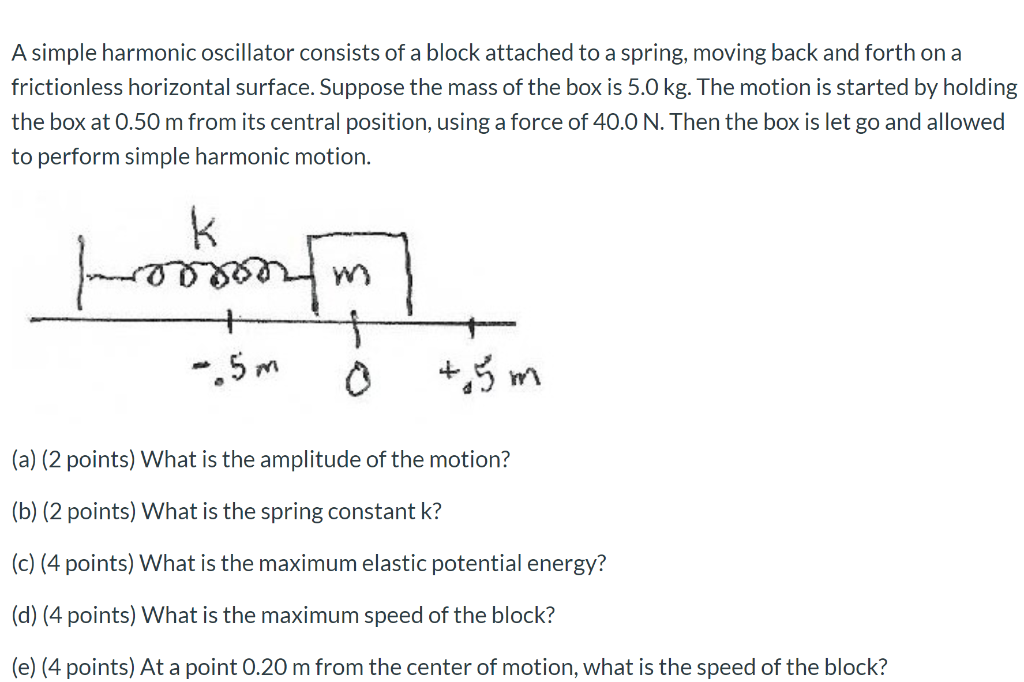A simple harmonic oscillator consists of a block attached to a spring, moving back and forth on a frictionless horizontal surface. Suppose the mass of the box is 5.0 kg. The motion is started by holding the box at 0.50 m from its central position, using a force of 40.0 N. Then the box is let go and allowed to perform simple harmonic motion. roosoo - 5m o +5 m (a) (2 points) What is the amplitude of the motion?...